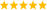# LabVIEW

cancel
Showing results for
Did you mean:

## measure percentage

Hello everyone! I'm doing on our project on labview. We are using a battery and need to measure the percentage of power that the battery left. As the power used, the voltage will also decrease. Here is a table of the relationship between voltage and percentage. Can anyone help me to get the vary value of percentage? Thanks!Message 1 of 43
(1,580 Views)

## Re: measure percentage

Are you having a problem with LabVIEW problem or a problem with math? What have you tried so far?LabVIEW Champion. It all comes together inGCentralMessage 2 of 43
(1,571 Views)

## Re: measure percentage

Both the problems...How to get the formula of the table? Then how to program on labview?

Message 3 of 43
(1,563 Views)

## Re: measure percentage

So what you mean here is, you want to display the exact percentage of battery depending on the voltage (Suppost 11.23 V mean 54% like that)? You can do a Linear fit (LinearFit.vi) with the Voltage as Y values and Percentage as X values (Or may be the other way) and find the Slope and Intercept. Then with the voltage reading Mulitply the slope and Add the intercept, you are done.

-----

The best solution is the one you find it by yourself
Message 4 of 43
(1,562 Views)

## Re: measure percentage

Create an array of points (cluster of xy pairs, x=voltage, y=%), then use interpolate array with the given voltage.LabVIEW Champion. It all comes together inGCentralMessage 5 of 43
(1,557 Views)

## Re: measure percentage

Actually it is not a linear one...

Message 6 of 43
(1,526 Views)

Maybe a curve.

Message 7 of 43
(1,525 Views)

## Re: measure percentage

Hi xuan,

either use Altenbach's suggestion (with linear interpolation between your data points) or calculate a polynomial regression of your choice. Then use PolynomialEvaluation function to calc battery state from voltage…

Best regards,
GerdW
CLAD expired, using LV2011SP1 + LV2017 (+LV2019 sometimes) on Win7+cRIO
Kudos are welcomeMessage 8 of 43
(1,514 Views)

## Re: measure percentage

Using a lookup table as Altenbach suggested is the easiest way to go.There are only two ways to tell somebody thanks: Kudos and Marked Solutions
Unofficial Forum Rules and Guidelines
Message 9 of 43
(1,497 Views)

## Re: measure percentage

I'm sorry...Can u give me a more detailed solution?

Message 10 of 43
(1,465 Views)